OUTPUT Statement
OUTPUT <OUT=SAS-data-set><options> ;

The OUTPUT statement creates a new SAS data set that contains all the variables in the input data set and, optionally, the estimated linear predictors and their standard error estimates, the estimates of the cumulative or individual response probabilities, and the confidence limits for the cumulative probabilities. Regression diagnostic statistics and estimates of cross validated response probabilities are also available for binary response models. If you specify more than one OUTPUT statement, only the last one is used. Formulas for the statistics are given in the sections Linear Predictor, Predicted Probability, and Confidence Limits and Regression Diagnostics, and, for conditional logistic regression, in the section Conditional Logistic Regression.

If you use the single-trial syntax, the data set also contains a variable named _LEVEL_, which indicates the level of the response that the given row of output is referring to. For instance, the value of the cumulative probability variable is the probability that the response variable is as large as the corresponding value of _LEVEL_. For details, see the section OUT= Output Data Set in the OUTPUT Statement.

The estimated linear predictor, its standard error estimate, all predicted probabilities, and the confidence limits for the cumulative probabilities are computed for all observations in which the explanatory variables have no missing values, even if the response is missing. By adding observations with missing response values to the input data set, you can compute these statistics for new observations or for settings of the explanatory variables not present in the data without affecting the model fit. Alternatively, the SCORE statement can be used to compute predicted probabilities and confidence intervals for new observations.

Table 53.8 lists the available options, which can be specified after a slash (/). The statistic and diagnostic options specify the statistics to be included in the output data set and name the new variables that contain the statistics. If a STRATA statement is specified, only the PREDICTED=, DFBETAS=, and H= options are available; see the section Regression Diagnostic Details for details.

Table 53.8 OUTPUT Statement Options

Option

Description

Specifiesfor the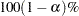confidence intervals

Names the output data set

Statistic Options

Names the lower confidence limit

PREDICTED=

Names the predicted probabilities

PREDPROBS=

Requests the individual, cumulative, or cross validated predicted probabilities

Names the standard error estimate of the linear predictor

Names the upper confidence limit

Names the linear predictor

Diagnostic Options for Binary Response

Names the confidence interval displacement

Names the confidence interval displacement

Names the standardized deletion parameter differences

Names the deletion chi-square goodness-of-fit change

Names the deletion deviance change

Names the leverage

Names the Pearson chi-square residual

Names the deviance residual

Names the likelihood residual

STDRESCHI=

Names the standardized Pearson chi-square residual

STDRESDEV=

Names the standardized deviance residual

The following list describes these options.

ALPHA=number

sets the level of significancefor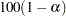% confidence limits for the appropriate response probabilities. The value of number must be between 0 and 1. By default, number is equal to the value of the ALPHA= option in the PROC LOGISTIC statement, or 0.05 if that option is not specified.

C=name

specifies the confidence interval displacement diagnostic that measures the influence of individual observations on the regression estimates.

CBAR=name

specifies the confidence interval displacement diagnostic that measures the overall change in the global regression estimates due to deleting an individual observation.

DFBETAS=_ALL_
DFBETAS=var-list

specifies the standardized differences in the regression estimates for assessing the effects of individual observations on the estimated regression parameters in the fitted model. You can specify a list of up to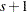variable names, whereis the number of explanatory variables in the MODEL statement, or you can specify just the keyword _ALL_. In the former specification, the first variable contains the standardized differences in the intercept estimate, the second variable contains the standardized differences in the parameter estimate for the first explanatory variable in the MODEL statement, and so on. In the latter specification, the DFBETAS statistics are named DFBETA_, whereis the name of the regression parameter. For example, if the model contains two variables X1 and X2, the specification DFBETAS=_ALL_ produces three DFBETAS statistics: DFBETA_Intercept, DFBETA_X1, and DFBETA_X2. If an explanatory variable is not included in the final model, the corresponding output variable named in DFBETAS=var-list contains missing values.

DIFCHISQ=name

specifies the change in the chi-square goodness-of-fit statistic attributable to deleting the individual observation.

DIFDEV=name

specifies the change in the deviance attributable to deleting the individual observation.

H=name

specifies the diagonal element of the hat matrix for detecting extreme points in the design space.

LOWER=name
L=name

names the variable containing the lower confidence limits for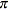, whereis the probability of the event response if events/trials syntax or single-trial syntax with binary response is specified; for a cumulative model,is cumulative probability (that is, the probability that the response is less than or equal to the value of _LEVEL_); for the generalized logit model, it is the individual probability (that is, the probability that the response category is represented by the value of _LEVEL_). See the ALPHA= option to set the confidence level.

OUT=SAS-data-set

names the output data set. If you omit the OUT= option, the output data set is created and given a default name by using the DATAconvention.

PREDICTED=name
PRED=name
PROB=name
P=name

names the variable containing the predicted probabilities. For the events/trials syntax or single-trial syntax with binary response, it is the predicted event probability. For a cumulative model, it is the predicted cumulative probability (that is, the probability that the response variable is less than or equal to the value of _LEVEL_); and for the generalized logit model, it is the predicted individual probability (that is, the probability of the response category represented by the value of _LEVEL_).

PREDPROBS=(keywords)

requests individual, cumulative, or cross validated predicted probabilities. Descriptions of the keywords are as follows.

INDIVIDUAL | I

requests the predicted probability of each response level. For a response variable Y with three levels, 1, 2, and 3, the individual probabilities are Pr(Y1), Pr(Y2), and Pr(Y3).

CUMULATIVE | C

requests the cumulative predicted probability of each response level. For a response variable Y with three levels, 1, 2, and 3, the cumulative probabilities are Pr(Y
1), Pr(Y2), and Pr(Y3). The cumulative probability for the last response level always has the constant value of 1. For generalized logit models, the cumulative predicted probabilities are not computed and are set to missing.

CROSSVALIDATE | XVALIDATE | X

requests the cross validated individual predicted probability of each response level. These probabilities are derived from the leave-one-out principle—that is, dropping the data of one subject and reestimating the parameter estimates. PROC LOGISTIC uses a less expensive one-step approximation to compute the parameter estimates. This option is valid only for binary response models; for nominal and ordinal models, the cross validated probabilities are not computed and are set to missing.

See the section Details of the PREDPROBS= Option at the end of this section for further details.

RESCHI=name

specifies the Pearson (chi-square) residual for identifying observations that are poorly accounted for by the model.

RESDEV=name

specifies the deviance residual for identifying poorly fitted observations.

RESLIK=name

specifies the likelihood residual for identifying poorly fitted observations.

STDRESCHI=name

specifies the standardized Pearson (chi-square) residual for identifying observations that are poorly accounted for by the model.

STDRESDEV=name

specifies the standardized deviance residual for identifying poorly fitted observations.

STDXBETA=name

names the variable containing the standard error estimates of XBETA. See the section Linear Predictor, Predicted Probability, and Confidence Limits for details.

UPPER=name
U=name

names the variable containing the upper confidence limits for, whereis the probability of the event response if events/trials syntax or single-trial syntax with binary response is specified; for a cumulative model,is cumulative probability (that is, the probability that the response is less than or equal to the value of _LEVEL_); for the generalized logit model, it is the individual probability (that is, the probability that the response category is represented by the value of _LEVEL_). See the ALPHA= option to set the confidence level.

XBETA=name

names the variable containing the estimates of the linear predictor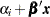, whereis the corresponding ordered value of _LEVEL_.

### Details of the PREDPROBS= Option

You can request any of the three types of predicted probabilities. For example, you can request both the individual predicted probabilities and the cross validated probabilities by specifying PREDPROBS=(I X).

When you specify the PREDPROBS= option, two automatic variables, _FROM_ and _INTO_, are included for the single-trial syntax and only one variable, _INTO_, is included for the events/trials syntax. The variable _FROM_ contains the formatted value of the observed response. The variable _INTO_ contains the formatted value of the response level with the largest individual predicted probability.

If you specify PREDPROBS=INDIVIDUAL, the OUT= data set containsadditional variables representing the individual probabilities, one for each response level, whereis the maximum number of response levels across all BY groups. The names of these variables have the form IP_xxx, where xxx represents the particular level. The representation depends on the following situations:

• If you specify events/trials syntax, xxx is either ‘Event’ or ‘Nonevent’. Thus, the variable containing the event probabilities is named IP_Event and the variable containing the nonevent probabilities is named IP_Nonevent.

• If you specify the single-trial syntax with more than one BY group, xxx is 1 for the first ordered level of the response, 2 for the second ordered level of the response, and so forth, as given in the "Response Profile" table. The variable containing the predicted probabilities Pr(Y=1) is named IP_1, where Y is the response variable. Similarly, IP_2 is the name of the variable containing the predicted probabilities Pr(Y=2), and so on.

• If you specify the single-trial syntax with no BY-group processing, xxx is the left-justified formatted value of the response level (the value might be truncated so that IP_xxx does not exceed 32 characters). For example, if Y is the response variable with response levels ‘None’, ‘Mild’, and ‘Severe’, the variables representing individual probabilities Pr(Y=’None’), P(Y=’Mild’), and P(Y=’Severe’) are named IP_None, IP_Mild, and IP_Severe, respectively.

If you specify PREDPROBS=CUMULATIVE, the OUT= data set containsadditional variables representing the cumulative probabilities, one for each response level, whereis the maximum number of response levels across all BY groups. The names of these variables have the form CP_xxx, where xxx represents the particular response level. The naming convention is similar to that given by PREDPROBS=INDIVIDUAL. The PREDPROBS=CUMULATIVE values are the same as those output by the PREDICT= option, but are arranged in variables on each output observation rather than in multiple output observations.

If you specify PREDPROBS=CROSSVALIDATE, the OUT= data set containsadditional variables representing the cross validated predicted probabilities of theresponse levels, whereis the maximum number of response levels across all BY groups. The names of these variables have the form XP_xxx, where xxx represents the particular level. The representation is the same as that given by PREDPROBS=INDIVIDUAL except that for the events/trials syntax there are four variables for the cross validated predicted probabilities instead of two:

XP_EVENT_R1E

is the cross validated predicted probability of an event when a current event trial is removed.

XP_NONEVENT_R1E

is the cross validated predicted probability of a nonevent when a current event trial is removed.

XP_EVENT_R1N

is the cross validated predicted probability of an event when a current nonevent trial is removed.

XP_NONEVENT_R1N

is the cross validated predicted probability of a nonevent when a current nonevent trial is removed.

The cross validated predicted probabilities are precisely those used in the CTABLE option. See the section Predicted Probability of an Event for Classification for details of the computation.